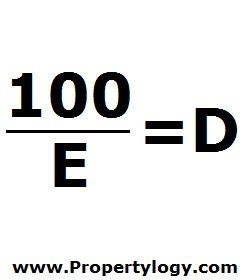Formula - Appraisal Depreciation | Propertylogy
Don't Miss

# Formula – Appraisal Depreciation

By on June 15, 2018

When appraisers calculate property value, they need to work out depreciation in order to work out a more accurate figure.

But depreciation meant for appraisal purposes is calculated differently from the typical depreciation meant for income reporting for tax returns.

This is the formula for appraisal depreciation100/E = D

E – Economic life

D – Annual depreciation

Appraisers need to determine the economic life of a property based on their expertise and experience.

The appraisal depreciation is expressed as a percentage.

If for example, a property has an economic age of 10 years, the when calculating the value of the property, depreciation (D) has to multiply by 10 and be deducted from the value.

Send this to a friend